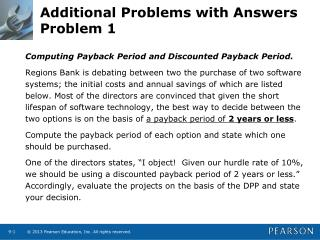DownloadDownload PresentationTélécharger la présentation- - - - - - - - - - - - - - - - - - - - - - - - - - - E N D - - - - - - - - - - - - - - - - - - - - - - - - - - -
##### Presentation Transcript

1. Additional Problems with AnswersProblem 1 Computing Payback Period and Discounted Payback Period. Regions Bank is debating between two the purchase of two software systems; the initial costs and annual savings of which are listed below. Most of the directors are convinced that given the short lifespan of software technology, the best way to decide between the two options is on the basis of a payback period of 2 years or less. Compute the payback period of each option and state which one should be purchased. One of the directors states, “I object! Given our hurdle rate of 10%, we should be using a discounted payback period of 2 years or less.” Accordingly, evaluate the projects on the basis of the DPP and state your decision.

2. Additional Problems with AnswersProblem 1 (Answer) Payback period of Option A = 1 year + (1,875,000-1,050,000)/900,000 = 1.92 years Payback period of Option B = 1year + (2,000,000-1,250,000)/800,000 = 1.9375 years. Based on the Payback Period, Option A should be chosen.

3. Additional Problems with AnswersProblem 1 (Answer) (continued) For the discounted payback period, we first discount the cash flows at 10% for the respective number of years and then add them up to see when we recover the investment. DPP A = -1,875,000 + 954,545.45+743,801.65=-176652.9  still to be recovered in Year 3  DPPA = 2 + (176652.9/338091.66) = 2.52 years DPP B = -2,000,000+1, 136,363.64+661157.02 = -202479.34 still to be recovered in Year 3 • DPPB = 2 + (202479.34/450788.88) = 2.45 years. Based on the Discounted Payback Period and a 2 year cutoff, neither option is acceptable.

4. Additional Problems with AnswersProblem 2 Computing Net Present Value – Independent projects: Locey Hardware Products is expanding its product line and its production capacity. The costs and expected cash flows of the two projects are given below. The firm typically uses a discount rate of 15.4 percent. • What are the NPVs of the two projects? • Which of the two projects should be accepted (if any) and why?

5. Additional Problems with AnswersProblem 2 (Answer) NPV @15.4% = \$86,572.61 \$20,736.91 Decision: Both NPVs are positive, and the projects are independent, so assuming that Locey Hardware has the required capital, both projects are acceptable.

6. Additional Problems with AnswersProblem 3 KLS Excavating needs a new crane. It has received two proposals from suppliers. Proposal A costs \$ 900,000 and generates cost savings of \$325,000 per year for 3 years, followed by savings of \$200,000 for an additional 2 years. Proposal B costs \$1,500,000 and generates cost savings of \$400,000 for 5 years. If KLS has a discount rate of 12%, and prefers using the IRR criterion to make investment decisions, which proposal should it accept?

8. Additional Problems with AnswersProblem 4 Using MIRR.   The New Performance Studio is looking to put on a new opera. They figure that the set-up and publicity will cost \$400,000. The show will go on for 3 years and bring in after-tax net cash flows of \$200,000 in Year 1; \$350,000 in Year 2; -\$50,000 in Year 3. If the firm has a required rate of return of 9% on its investments, evaluate whether the show should go on using the MIRR approach.

9. Additional Problems with AnswersProblem 4 (Answer) The forecasted after-tax net cash flows are as follows:  Year After-tax cash flow 0 -\$400,000 1 200,000 2 350,000 3 -\$50,000 The formula for MIRR is as follows: Where : FV = Compounded value of cash inflows at end of project’s life (Year 3)using realistic reinvestment rate (9%); PV = Discounted value of all cash outflows at Year 0; N = number of years until the end of the project’s life= 3.

10. Additional Problems with AnswersProblem 4 (Answer) (continued) FV3 = \$200,000*(1.09)2 + \$350,000*(1.09)1 = \$237,620 + \$381,500 = \$619,120 PV0 = \$400,000 + \$50,000/(1.09)3 =\$438,609.17 MIRR = (619,120/\$438,609.17)1/3 – 1 = (1.411552)1/3 -1 = 12.18% The show must go on, since the MIRR = 12.18% > Hurdle rate = 9%